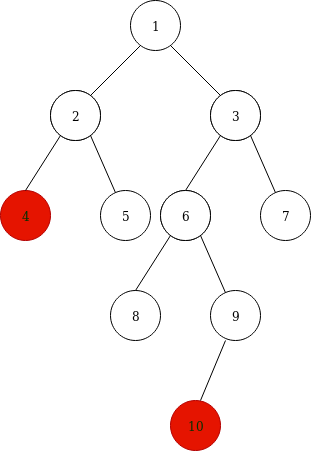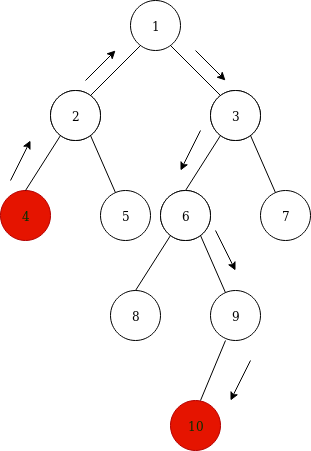### C++ program to print the longest leaf to leaf path in a binary tree

Longest leaf to leaf path in a binary tree, in other words, the diameter path of a binary tree.
The diameter of a tree is the number of nodes between the two farthest nodes in the tree.
Two farthest nodes have been highlighted.The diameter path is highlighted with arrowheads. A binary tree may have multiple diameter paths.To print the longest leaf to leaf path first, we find the height of the binary tree, then the diameter and finally we traverse through the nodes and print the path which has the height of tree. First, the left subtree path is printed in the reverse order, then the root node of diameter path, then the right subtree path.

The below program will print one of the longest leaf to leaf paths if multiple paths are present.

Input Tree

//            1
//         /    \
//        2      3
//      /   \   /  \
//     4     5  6   7
//             /  \
//            8     9
//                 /
//                10

Output :

Longest leaf to leaf path : 4 2 1 3 6 9 10
Diameter is 7

Program:

```#include <iostream>

using namespace std;

//Tree structure
struct Node
{
int data;
Node *left, *right;
};

class CalculationBinaryTree
{
public:

//Create new Node
struct Node* newNode(int data)
{
struct Node* node = new Node;
node->data = data;
node->left = node->right = NULL;

return (node);
}
//To find the tree height
int height(Node *root, int& diameter, Node* &tempRoot, int& leftHeight, int& rightHeight, int& flag)
{
if(root == NULL)
return 0;

int left_height = height(root->left, diameter, tempRoot, leftHeight, rightHeight, flag);
int right_height = height(root->right, diameter, tempRoot, leftHeight, rightHeight, flag);

if(diameter < 1 + left_height + right_height)
{
diameter = 1 + left_height + right_height;

//Store root, left and right tree heights to print the path
tempRoot = root;
leftHeight = left_height;
rightHeight = right_height;
}

return 1 + max(left_height, right_height);
}

void printPath(int nodes[], int length, int flag)
{
int  i;

//Flag = 0 => to print the left path in reverse order
if(flag == 0)
{
for(i = length -1; i >= 0; i--)
{
cout<<"\t"<<nodes[i];
}
}
//To print the right path
else if(flag == 1)
{
for(i = 0; i < length; i++)
{
cout<<"\t"<<nodes[i];
}
}
}

//To recursively check the node path which is equal to the height
void checkPath(Node* node, int nodes[], int pathLen, int maxHeight, int& flag)
{
if (node == NULL)
return;

// store the node in path array
nodes[pathLen] = node->data;
pathLen++;

// If leaf, then print the path that led to the leaf
if (node->left == NULL && node->right == NULL)
{

// print path which is equal to height of the tree.
if (pathLen == maxHeight && (flag == 0 || flag == 1))
{
printPath(nodes, pathLen, flag);
flag = 2;
}
}

else
{

// otherwise check both subtrees
checkPath(node->left, nodes, pathLen, maxHeight, flag);
checkPath(node->right, nodes, pathLen, maxHeight, flag);
}
}
int diameter(Node *root)
{
if(root ==  NULL)
return 0;

int diameter = -1;
int flag = 0, leftHeight = 0, rightHeight = 0, pathLength = 0, leftPath;

Node *tempRoot;

int tree_height = height(root, diameter, tempRoot, leftHeight, rightHeight, flag);

checkPath(tempRoot->left, leftPath, pathLength, leftHeight, flag);
//Print root of diamater path

cout<<"\t"<<tempRoot->data;
int rightPath;
flag = 1;

checkPath(tempRoot->right, rightPath, pathLength, rightHeight, flag);
return diameter;
}
};

int main()
{
CalculationBinaryTree obj ;

struct Node* root =  obj.newNode(1);

root->left = obj.newNode(2);
root->right =  obj.newNode(3);
root->left->left = obj.newNode(4);
root->left->right = obj.newNode(5);
root->right->left = obj.newNode(6);
root->right->right = obj.newNode(7);
root->right->left->left = obj.newNode(8);
root->right->left->right = obj.newNode(9);
root->right->left->right->left = obj.newNode(10);

//            1
//         /    \
//        2      3
//      /   \   /  \
//     4     5  6   7
//             /  \
//            8     9
//                 /
//                10
cout<<"\n Longest leaf to leaf path : ";
int diameter =  obj.diameter(root);

cout<<"\n Diameter is "<<diameter<<"\n";
return 0;
}```